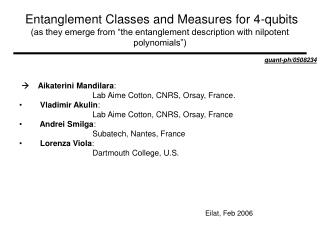DownloadDownload Presentationquant-ph/0508234  Aikaterini Mandilara :

# quant-ph/0508234  Aikaterini Mandilara :

Télécharger la présentation## quant-ph/0508234  Aikaterini Mandilara :

- - - - - - - - - - - - - - - - - - - - - - - - - - - E N D - - - - - - - - - - - - - - - - - - - - - - - - - - -
##### Presentation Transcript

1. Entanglement Classes and Measures for 4-qubits(as they emerge from “the entanglement description with nilpotent polynomials”) quant-ph/0508234  Aikaterini Mandilara: Lab Aime Cotton, CNRS, Orsay, France. • Vladimir Akulin: Lab Aime Cotton, CNRS, Orsay, France • Andrei Smilga: Subatech, Nantes, France • Lorenza Viola: Dartmouth College, U.S. Eilat, Feb 2006

2. Outline: • Writing a quantum state as a nilpotent polynomial. Nilpotential. Tanglemeter. • Entanglement classes (sl-orbits) sl-tanglemeter. • Entanglement measures  coefficients of the tanglemeter. • Conclusions. Open questions. Su-orbit

3. From quantum states to nilpotential + Nilpotential: Extensive property: Product states become sum Dynamics:

4. From nilpotential to tanglemeter 1 2 3 4 …. n A state/nilpotential of N qubits An orbit of states All the states in the orbit Should have the same Entanglement description …. SU(2) SU(2) SU(2) SU(2) …… SU(2) 3 parameters each one How many parameters for the orbit marker? Tanglemeter Physical condition: Maximize Method: use feedback in dynamical equations

5. More general, non-unitary, reversible, local operations • nonselective LOCC operations= local operations assisted by classical communication • selective SLOCC= stochastic LOCC (Bennet et al, PRA 63, 012307) Indirect measurement a s *If ignore the normalization & divide by det(M): SLOCC described by SL(2,C) generators: Entanglement Classes = set of states which are equivalent under local SLOCC operations • Three qubits can be entangled in two inequivalent ways : W. Dur etal,PRA 62, 062314, (2000) • Four qubits can be entangled in nine different ways: F. Verstraete et al, PRA 65: 052112 (2002).

6. sl-tanglemeterEntanglement Classes 1 2 3 4 …. n A state/nilpotential of N qubits An sl-orbit of states Merging different su-orbits together. …. SL(2,C) SL(2,C) SL(2,C) SL(2,C) .. SL(2,C) 6 parameters each one How many parameters for the orbit marker? In general.. Sl-Tanglemeter.. sl-orbit marker Physical condition:? Method: use feedback in dynamical equations

7. su orbits tanglemeter= su-orbit marker sl orbits (entanglement classes) sl-tanglemeter= sl-orbit marker 2 qubits 3 qubits A. Miyake 03 4 qubits family of general orbits

8. Entanglement Measures In order to compare different su-orbits in the same sl-orbit or different sl-orbits in the same general family of orbits SU- Measures SL-Measures • (Give 0 for separable state) and 1 for maximally entangled state of the sl-orbit • Invariant under local • SU operations and nonincreasing • under LOCC transformations • Give 1 for the maximally entangled state of the family of the sl-orbits • Invariant under local • SLOCC operations Polynomial invariants on the amplitudes of the states 2 ways to construct invariants: Invariant coefficients of the tanglemeter But, which su-invariants are decreasing under LOCC? The poly-inv. which are sl-invariants

9. sl-tanglemeter for 4 qubits SU- Measures SL-Measures Polynomial invariants: Only to be used in the states Belonging to the states above Tanglemeter’s coefficients: • We start we the normalized state • We apply sl-transformations to put in the sl-canonic form. • The normalization of the state • give us a measure on nonunitarity/distance of the • Initial state to the maximal entangled state.

10. Conclusions: • With sl-tanglemeter we can at least identify the most general class of entanglement for N qubits. It can be generalized to ensembles of quDits. • Investigate a little bit more in the special classes and their applications. • We introduced the idea of sl-invariant measures that extends the idea of su-measures. • Tanglemeter’s coefficients can serve as invariants for construction of measures.

11. Acknowledgements • My advisor in WashU: J. W. Clark • The coworkers on this project: V. M. Akulin A. V. Smilga Lorenza Viola • Prof. G. Kurizki